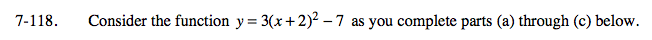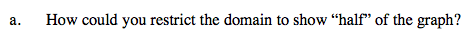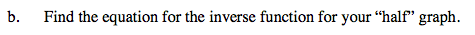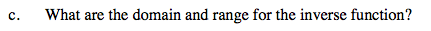Home > A2C > Chapter 7 > Lesson 7.2.2 > Problem7-118

7-118.
1. Consider the function y = 3(x + 2)2 − 7 as you complete parts (a) through (c) below. Homework Help ✎

1. How could you restrict the domain to show “half” of the graph?

2. Find the equation for the inverse function for your “half” graph.

3. What are the domain and range for the inverse function?Where is the line of symmetry?Start by finding an inverse equation. Then consider the domain that you chose.Remember the relationship between the domain and range of the original and the domain and range of the inverse.